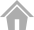# ANEO Sponsored Puzzle discussion

#90

I had different results when used float and double types in java.
This task good for static type languages i think.

#91

90 is in km/h
3000 is in m
30 is in s

so, first convert 90km/h -> 25 m/s

so now: 25*30 = 750m we have just crossed the first light…

#92

I really wonder : is there a mathematical answer to this problem ?
Every attempt to work around rounding problems seems not very good.

#93

Failed test case 2, it was expecting 36 even though the max speed was 50. Seemed broken, so I submitted my code and then it passed test case 2 on submission.

I think something is broken with this puzzle.

#94

Hi! I just solved the problem, using the number theory approach in less than 20 lines of code. The integers went way high, but Python can handle large integers well. Writing down the equations helps to see that the velocity conversion between [km/h] and [m/s] is actually not necessary. This discrepancy of dimensions can be conquered by other means.

#95

Scala or Java for my part. But also tried using Python3

#96

apart from my recurring mistake of thinking the distances given in the input data were relative (while they are not, they’re all absolute), i had to change my strategy once to make it. at first i was thinking in a simulation fashion, but it didn’t seem to agree with the creators’ algorithm and i was stumped. i still wonder if i made errors in my logic, or if it was precision issues, but one thing for sure, it didn’t work. thinking in terms of each light being a step in the algorithm allowed me to avoid using floating numbers as accumulators and i guess i matched the original algorithm. phew!

#97

I’m really interested by those ohter means. I can’t figure out how to solve this puzzle without converting km/h to m/s. Could you give some hints or links to some ressources explaining how to do ? Thx

#98

instead of “simulating” movement, like, adding a certain floating point number of meters each second using km/h -> m/s conversion, think backwards: how many seconds were elapsed once the car has reached the first light, then the second light, etc. this allows to keep all “accumulators” as integers!

#99

the most important information is, that the distances are absolute and not relative.

#100

Maybe somebody will help.
I have wrong understood this:
All traffic lights turn green at the same time as you enter the area.
Not all ligths turn green at one time at start, but at specific time, when you enter the every area and turn at different time. the same comment is above.

#101

But how can you find a number of seconds without converting your initial speed expressed in km/h ? A conversion is unavoidable and floating point rounding problem equally.

#102

you have to think of the problem the other way around. instead of “stepping” through seconds and accumulate floating point numbers of kilometers or meters (which is how i coded my solution to the problem at first as well), step through kilometers and find out how much time this equals to. i don’t know if this is too much hint to give in here, but i feel like that’s the core of the problem! it should’ve been possible to do it the other way around, but they made the problem so we have to be precise to a greater point than accumulating floating point values can, or they just wanted us to find the exact algorithm they used.

#103

is it possible to do 100% with js? does someone do it? I use, conversions, rounds, multiplications …

#104

You have to deal with doubles appromixations. I use a very dirty `+ 0.1` in my code#105

i have no idea for it , can you give me the answer for example in python?

#106

Of course we won’t.

#107

In ruby then ?#108

Convert km/h to m/s to avoid floating point errors.

#109

Great puzzle. Enjoyed it!